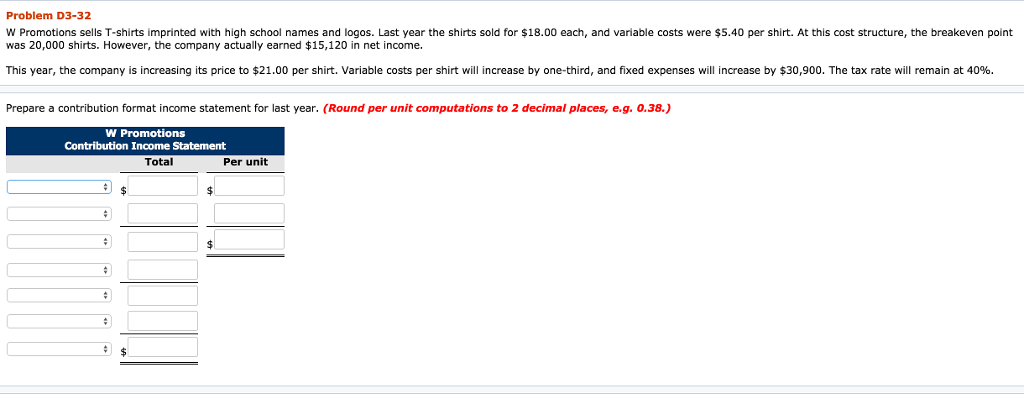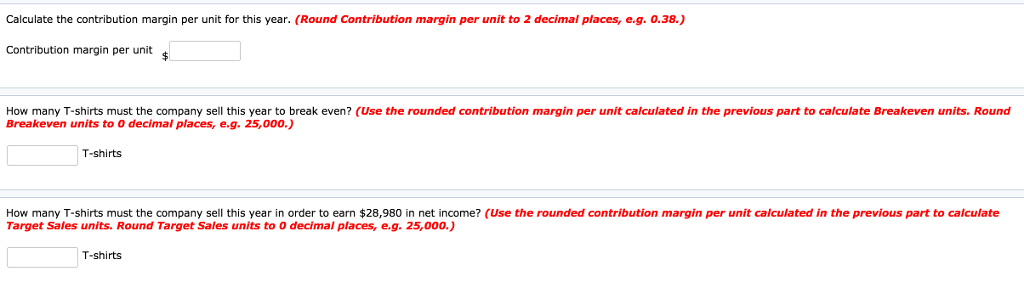# Question & Answer: Problem D3-32 W Promotions sells T-shirts imprinted with high school names and logos. Last year the shirts sold for \$18.00 ea…..Problem D3-32 W Promotions sells T-shirts imprinted with high school names and logos. Last year the shirts sold for \$18.00 each, and variable costs were \$5.40 per shirt. At this cost structure, the breakeven point was 20,000 shirts. However, the company actually earned \$15,120 in net income. This year, the company is increasing its price to \$21.00 per shirt. Variable costs per shirt will increase by one-third, and fixed expenses will increase by \$30 900 The tax rate will remain at 40% Prepare a contribution format income statement for last year. (Round per unit computations to 2 decimal places, e.g. 0.38.) Contribution Income Statement Total Per unit

Don't use plagiarized sources. Get Your Custom Essay on
Question & Answer: Problem D3-32 W Promotions sells T-shirts imprinted with high school names and logos. Last year the shirts sold for \$18.00 ea…..
GET AN ESSAY WRITTEN FOR YOU FROM AS LOW AS \$13/PAGE

Solution:

Per unit

 Sale price \$18 Variable cost \$5.40 Contribution per unit \$12.60 Less fixed cost Profit Less tax(40%) Net income

Break even point(units)=fixed cost/contribution per unit

20000 unit=fixed cost/\$12.60

Fixed cost=\$252,000

Tax=(\$15,120/.60)×.40

=\$10,080

Profit=net income+tax

=15,120+10,080

=\$25,200

Contribution=Profit+fixed cost

=\$25,200+252,000

=\$277,200

Contribution per unit=contribution/unit sold

\$12.60=\$277,200/unit sold

Unit sold=22,000 units

1. Contribution format income statement:

 Total per unit Sales(22000 units) \$396,000 \$18 Less variable cost \$118,800 \$5.40 Contribution \$277,200 \$12.60 Less fixed cost \$252,000 Profit \$25,200 Less tax(40%) \$10,080 Net income \$15,120

2.

New sales price per unit=\$21

New variable cost (\$5.40+(5.40×1/3)]=\$7.2

New contribution margin per unit=\$13.8

3. This year break even:

=New fixed cost/new contribution margin per unit

=(\$252,000+30,900)/\$13.80

=20,500 units

4.

Desired before tax profit=\$28980/.60

=\$48,300

Break even units to get desired profit=( new fixed cost+desired profit)/contribution margin per unit

=(252000+30,900+48,300)/\$13.80

=24,000 units